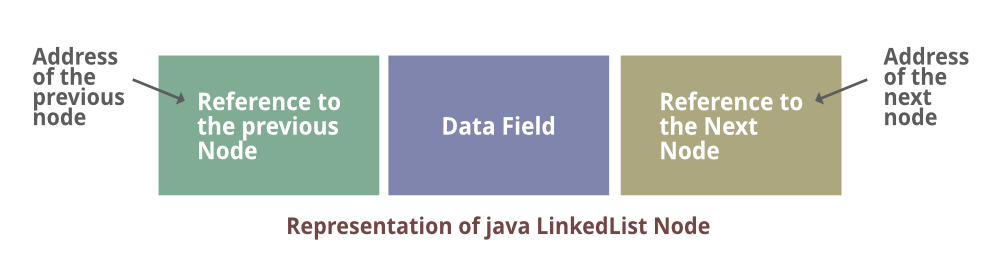GeeksforGeeks App
Open AppBrowser
Continue

# XOR Linked List – A Memory Efficient Doubly Linked List | Set 1

An ordinary Doubly Linked List requires space for two address fields to store the addresses of previous and next nodes. It is represented as follows in the image below. From the below image, it can be depicted out that the address of the previous node is retained and carried over for computation by the previous pointer while that of the next node is after pointers similarly.Now there is a memory-efficient version of Doubly Linked List that can be created using only one space for the address field with every node. This memory efficient Doubly Linked List is called XOR Linked List or Memory Efficient as the list uses bitwise XOR operation to save space for one address. In the XOR linked list, instead of storing actual memory addresses, every node stores the XOR of addresses of previous and next nodes.

Consider the above Doubly Linked List. Following are the Ordinary and XOR (or Memory Efficient) representations of the Doubly Linked List.Now here we will be discussing out both the ways in order to perch out how XOR representation behaves differently from ordinary representation.

1. Ordinary Representation
2. XOR List Representation

Way 1: Ordinary Representation

Node A:

prev = NULL, next = add(B) // previous is NULL and next is address of B

Node B:

Node C:

Node D:

prev = add(C), next = NULL // previous is address of C and next is NULL

Way 2: XOR List Representation

Let us call the address variable in XOR representation npx (XOR of next and previous)

While traversing XOR Linked List we can traverse the XOR list in both forward and reverse directions. While traversing the list we need to remember the address of the previously accessed node in order to calculate the next node’s address.

For example: When we are at node C, we must have the address of B. XOR of add(B) and npx of C gives us the add(D).

Illustration:

Node A:

npx = 0 XOR add(B) // bitwise XOR of zero and address of B

Node B:

Node C:

Node D:

npx = add(C) XOR 0 // bitwise XOR of address of C and 0

```npx(C) XOR add(B)
=> add(D) XOR 0  // a^a = 0
=> add(D)     // a^0 = a```

Similarly, we can traverse the list in the backward direction. Now straightaway coming down to the implementation part in order to figure out better.

Below is the implementation of the above approach:

## C++

 `// C++ Implementation of Memory``// efficient Doubly Linked List`` ` `// Importing libraries``#include ``#include `` ` `using` `namespace` `std;`` ` `// Class 1``// Helper class(Node structure)``class` `Node {``    ``public` `: ``int` `data;``    ``// Xor of next node and previous node``    ``Node* xnode;``};`` ` `// Method 1``// It returns Xored value of the node addresses``Node* Xor(Node* x, Node* y)``{``    ``return` `reinterpret_cast``(``        ``reinterpret_cast``<``uintptr_t``>(x)``        ``^ ``reinterpret_cast``<``uintptr_t``>(y));``}`` ` `// Method 2``// Insert a node at the start of the Xored LinkedList and``// mark the newly inserted node as head``void` `insert(Node** head_ref, ``int` `data)``{``    ``// Allocate memory for new node``    ``Node* new_node = ``new` `Node();``    ``new_node -> data = data;`` ` `    ``// Since new node is inserted at the``    ``// start , xnode of new node will always be``    ``// Xor of current head and NULL``    ``new_node -> xnode = *head_ref;`` ` `    ``// If linkedlist is not empty, then xnode of``    ``// present head node will be Xor of new node``    ``// and node next to current head */``    ``if` `(*head_ref != NULL) {``        ``// *(head_ref)->xnode is Xor of (NULL and next).``        ``// If we Xor Null with next we get next``        ``(*head_ref)``            ``-> xnode = Xor(new_node, (*head_ref) -> xnode);``    ``}`` ` `    ``// Change head``    ``*head_ref = new_node;``}`` ` `// Method 3``// It simply prints contents of doubly linked``// list in forward direction``void` `printList(Node* head)``{``    ``Node* curr = head;``    ``Node* prev = NULL;``    ``Node* next;`` ` `    ``cout << ``"The nodes of Linked List are: \n"``;`` ` `    ``// Till condition holds true``    ``while` `(curr != NULL) {``        ``// print current node``        ``cout << curr -> data << ``" "``;`` ` `        ``// get address of next node: curr->xnode is``        ``// next^prev, so curr->xnode^prev will be``        ``// next^prev^prev which is next``        ``next = Xor(prev, curr -> xnode);`` ` `        ``// update prev and curr for next iteration``        ``prev = curr;``        ``curr = next;``    ``}``}`` ` `// Method 4``// main driver method``int` `main()``{``    ``Node* head = NULL;``    ``insert(&head, 10);``    ``insert(&head, 100);``    ``insert(&head, 1000);``    ``insert(&head, 10000);`` ` `    ``// Printing the created list``    ``printList(head);`` ` `    ``return` `(0);``}`

Output

```The nodes of Linked List are:
10000 1000 100 10 ```

Time Complexity: O(n)
Auxiliary Space: O(1)

My Personal Notes arrow_drop_up